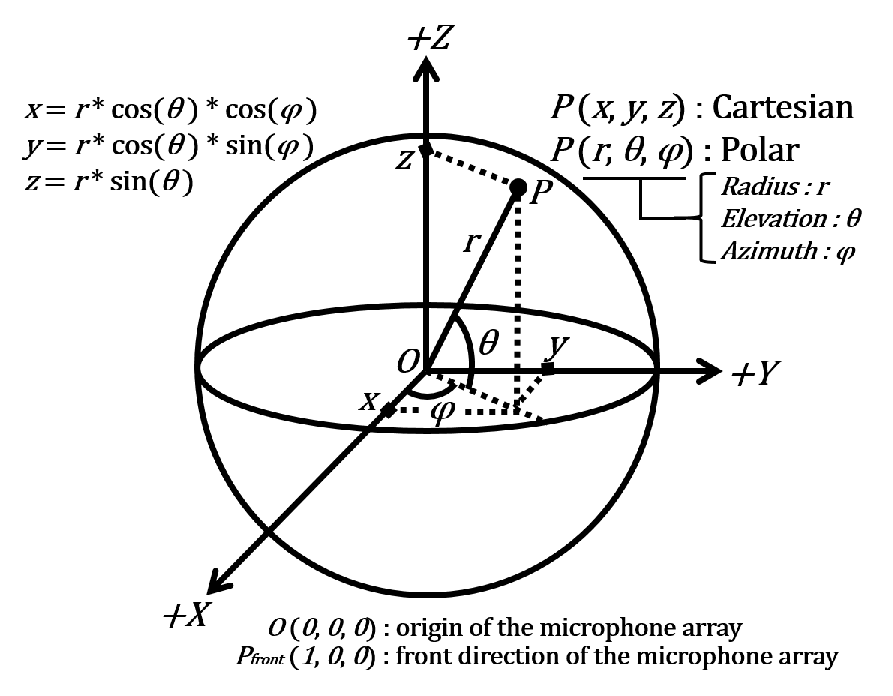# 4.5 HARK Standard Coordinate System

The center of HARK  ’s coordinate system is the origin specified by the user (usually the center of the microphone array). The x positive axis is the front, y positive axis is towards the left, and z positive axis is in the upward direction. The unit is in meters. It is assumed that the front angle is 0[deg] and is rotating in a counterclockwise direction (ex: left direction is 90[deg]). The angle of elevation is defined as the XY plane and formed angle of the direction vector of the coordinates.Figure 4.2: HARK standard coordinate system